# Precalculus : Find the Critical Numbers of a Function

## Example Questions

2 Next →

### Example Question #11 : Find The Critical Numbers Of A Function

Find the critical value(s) of a function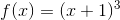.

Possible Answers: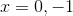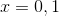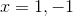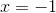Correct answer:Explanation:

The critical values of a functionare values for which the derivative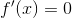. In this case: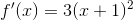Setting: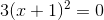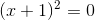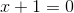### Example Question #12 : Find The Critical Numbers Of A Function

Find the critical numbers of the following function: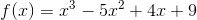Possible Answers: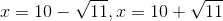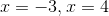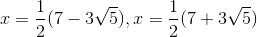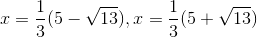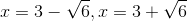Correct answer:Explanation:

The critical numbers of a function are the points at which its slope is zero, which tells where the function has a minimum or maximum. The slope of a function is described by its derivative, so we'll take the derivative of the function and set it equal to 0 to solve for the x values of the critical numbers: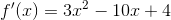It appears the derivative cannot be factored to solve for x, so we'll have to use the quadratic formula to find the critical numbers: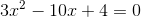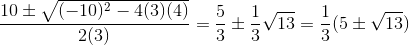So the critical numbers occur at the following two values of x:### Example Question #13 : Find The Critical Numbers Of A Function

Given the following function, find the critical numbers: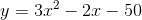Possible Answers: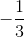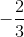Correct answer:Explanation:

Critical numbers are where the slope of the function is equal to zero or undefined.

Find the derivative and set the derivative function to zero.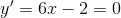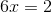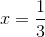There is only one critical value at.

### Example Question #14 : Find The Critical Numbers Of A Function

Determine the critical numbers of the functionPossible Answers: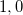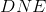Correct answer:Explanation:

The critical numbers are thevalues for which either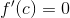or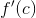is undefined.

In order to find the first derivative we use the power rule which states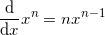Applying this rule term by term we get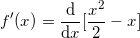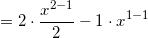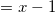The first derivative is defined for all values of x. Setting the first derivative to zero yields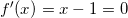As such, the critical number is### Example Question #15 : Find The Critical Numbers Of A Function

Find the critical values of the following function: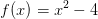Possible Answers: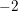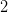Correct answer:Explanation:

To solve, simply find the first derivative and find when it is equal to 0. To find the first derivative, we must use the power rule as outlind below.

Power rule: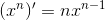Thus,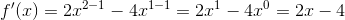Now, we must set out function equal to 0 and solve for x. Thus,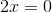Dividing both sides by 2, we get:### Example Question #11 : Find The Critical Numbers Of A Function

Find the critical values of the following function.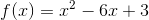Possible Answers: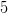Correct answer:Explanation:

To solve, simply differentiate using the power rule, as outlined below.

Power rule states,.

Thus given,our first derivative is: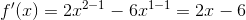Then plug in 0 for f(x) to find when our function is equal to 0.

Thus,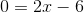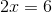2 Next →

### All Precalculus Resources